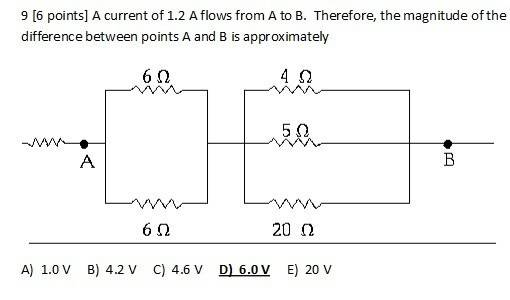# Magnitude of Potential Difference Between Two Points1. Homework Statement [/b]

So I found this problem online, and I really do not know how to go about solving it! My professor has done similar problems in class, but it's still a little confusing.

A current of 1.2 A flows from A to B. Therefore, the magnitude of the potential difference between points A and B is approximately

## Homework Equations

I know Δv=IR and Ampere/Volt makes an Ω

## The Attempt at a Solution

Well, the image attached already gives the answer, it should be 6.0 V. The current is given (1.2 A), so I'm guessing by the units that I must multiply something with Ω to get Volts. Should I add up the resistors?

Thank you!

Last edited:

lewando
Homework Helper
Gold Member
To do this problem, you are going to need to be able to "add" resistors in parallel and series. Need help with that?

gneill
Mentor
Well, the image attached already gives the answer, it should be 6.0 V. The current is given (1.2 A), so I'm guessing by the units that I must multiply something with Ω to get Volts. Should I add up the resistors?

Sure. Find the equivalent resistance between points A and B. Then see what potential drop occurs across it due to the given current value.

To do this problem, you are going to need to be able to "add" resistors in parallel and series. Need help with that?